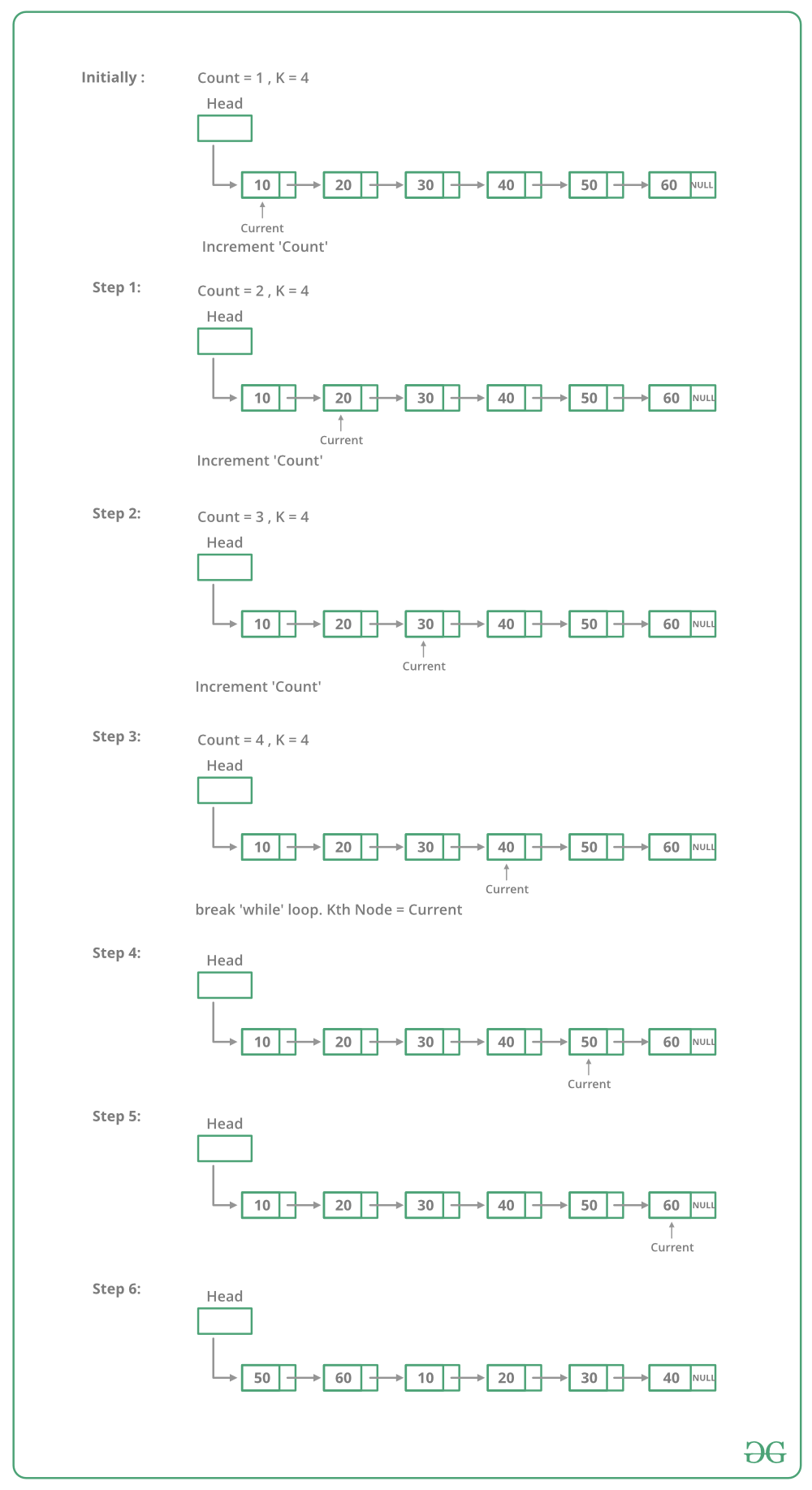Open In App

# Python Program For Rotating A Linked List

Given a singly linked list, rotate the linked list counter-clockwise by k nodes. Where k is a given positive integer. For example, if the given linked list is 10->20->30->40->50->60 and k is 4, the list should be modified to 50->60->10->20->30->40. Assume that k is smaller than the count of nodes in a linked list.

Method 1:
To rotate the linked list, we need to change the next of kth node to NULL, the next of the last node to the previous head node, and finally, change the head to (k+1)th node. So we need to get hold of three nodes: kth node, (k+1)th node, and last node.
Traverse the list from the beginning and stop at kth node. Store pointer to kth node. We can get (k+1)th node using kthNode->next. Keep traversing till the end and store a pointer to the last node also. Finally, change pointers as stated above.

Below image shows how to rotate function works in the code :## Python

 `# Python program to rotate ``# a linked list``# Node class ``class` `Node:`` ` `    ``# Constructor to initialize ``    ``# the node object``    ``def` `__init__(``self``, data):``        ``self``.data ``=` `data``        ``self``.``next` `=` `None`` ` `class` `LinkedList:`` ` `    ``# Function to initialize head``    ``def` `__init__(``self``):``        ``self``.head ``=` `None`` ` `    ``# Function to insert a new node``    ``# at the beginning``    ``def` `push(``self``, new_data):``        ``# allocate node and put the data``        ``new_node ``=` `Node(new_data)`` ` `        ``# Make next of new node as head``        ``new_node.``next` `=` `self``.head``         ` `        ``# Move the head to point to the ``        ``# new Node``        ``self``.head ``=` `new_node`` ` `    ``# Utility function to print it the ``    ``# linked LinkedList``    ``def` `printList(``self``):``        ``temp ``=` `self``.head``        ``while``(temp):``            ``print` `temp.data,``            ``temp ``=` `temp.``next`` ` `    ``# This function rotates a linked list ``    ``# counter-clockwise and updates the ``    ``# head. The function assumes that k ``    ``# is smaller than size of linked list. ``    ``# It doesn't modify the list if k is ``    ``# greater than of equal to size``    ``def` `rotate(``self``, k):``        ``if` `k ``=``=` `0``: ``            ``return` `         ` `        ``# Let us understand the below code ``        ``# for example k = 4 and list = ``        ``# 10->20->30->40->50->60``        ``current ``=` `self``.head``         ` `        ``# current will either point to kth ``        ``# or NULL after this loop current ``        ``# will point to node 40 in the above ``        ``# example``        ``count ``=` `1` `        ``while``(count 20->30->40->50->60``for` `i ``in` `range``(``60``, ``0``, ``-``10``):``    ``llist.push(i)`` ` `print` `"Given linked list"``llist.printList()``llist.rotate(``4``)`` ` `print` `"Rotated Linked list"``llist.printList()``# This code is contributed by Nikhil Kumar Singh(nickzuck_007)`

p>Output:

```Given linked list
10  20  30  40  50  60
50  60  10  20  30  40```

Time Complexity: O(n) where n is the number of nodes in Linked List. The code traverses the linked list only once.

Method 2:
To rotate a linked list by k, we can first make the linked list circular and then moving k-1 steps forward from head node, making (k-1)th node’s next to null and make kth node as head.

## Python3

 `# Python3 program to rotate a ``# linked list counter clock wise``  ` `# Link list node ``class` `Node:``     ` `    ``def` `__init__(``self``):``         ` `        ``self``.data ``=` `0``        ``self``.``next` `=` `None`` ` `# This function rotates a linked list``# counter-clockwise and updates the``# head. The function assumes that k is``# smaller than size of linked list.``def` `rotate(head_ref, k):`` ` `    ``if` `(k ``=``=` `0``):``        ``return``  ` `    ``# Let us understand the below``    ``# code for example k = 4 and``    ``# list = 10.20.30.40.50.60.``    ``current ``=` `head_ref``  ` `    ``# Traverse till the end.``    ``while` `(current.``next` `!``=` `None``):``        ``current ``=` `current.``next``  ` `    ``current.``next` `=` `head_ref``    ``current ``=` `head_ref``     ` `    ``# Traverse the linked list to k-1 ``    ``# position which will be last ``    ``# element for rotated array.``    ``for` `i ``in` `range``(k ``-` `1``):``        ``current ``=` `current.``next``  ` `    ``# Update the head_ref and last ``    ``# element pointer to None``    ``head_ref ``=` `current.``next``    ``current.``next` `=` `None``    ``return` `head_ref``  ` `# UTILITY FUNCTIONS ``# Function to push a node ``def` `push(head_ref, new_data):`` ` `    ``# Allocate node ``    ``new_node ``=` `Node()``  ` `    ``# Put in the data ``    ``new_node.data ``=` `new_data``  ` `    ``# Link the old list off ``    ``# the new node ``    ``new_node.``next` `=` `(head_ref)``  ` `    ``# Move the head to point``    ``# to the new node ``    ``(head_ref) ``=` `new_node``    ``return` `head_ref``     ` `# Function to print linked list ``def` `printList(node):`` ` `    ``while` `(node !``=` `None``):``        ``print``(node.data, end ``=` `' '``)``        ``node ``=` `node.``next`` ` `# Driver code``if` `__name__``=``=``'__main__'``:``     ` `    ``# Start with the empty list ``    ``head ``=` `None``  ` `    ``# Create a list ``    ``# 10.20.30.40.50.60``    ``for` `i ``in` `range``(``60``, ``0``, ``-``10``):``        ``head ``=` `push(head, i)``  ` `    ``print``(``"Given linked list "``)``    ``printList(head)``    ``head ``=` `rotate(head, ``4``)``  ` `    ``print``(``"\nRotated Linked list "``)``    ``printList(head)``# This code is contributed by rutvik_56`

Output:

```Given linked list
10 20 30 40 50 60